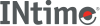﻿ hypotINtime SDK Help
hypot

Calculates the Euclidean distance function.

```#include <math.h>

double hypot(double x, double y);
```

#### Remarks

These functions shall compute the value of the square root of `x`2+ `y`2 without undue overflow or underflow.

An application wishing to check for error situations should set errno to zero and call feclearexcept(FE_ALL_EXCEPT) before calling these functions. On return, if errno is non-zero or fetestexcept (FE_INVALID | FE_DIVBYZERO | FE_OVERFLOW | FE_UNDERFLOW) is non-zero, an error has occurred.

#### Return Values

• Upon successful completion, these functions shall return the length of the hypotenuse of a right-angled triangle with sides of length `x` and `y`.
• If the correct value would cause overflow, a range error shall occur and hypot(), hypotf(), and hypotl() shall return the value of the macro HUGE_VAL, HUGE_VALF, and HUGE_VALL, respectively.
• If `x` or `y` is -Inf, +Inf shall be returned (even if one of `x` or `y` is NaN).
• If `x` or `y` is NaN, and the other is not -Inf, a NaN shall be returned.
• If both arguments are subnormal and the correct result is subnormal, a range error may occur and the correct result is returned.

#### Requirements

Versions Defined in Include Link to
INtime 3.0 intime/rt/include/math.h math.h clib.lib